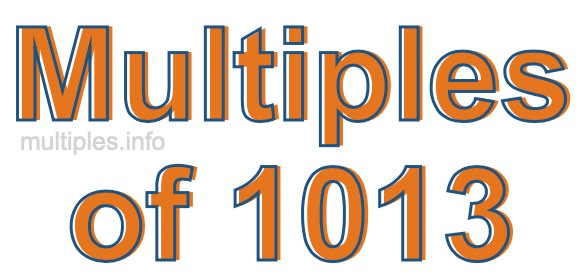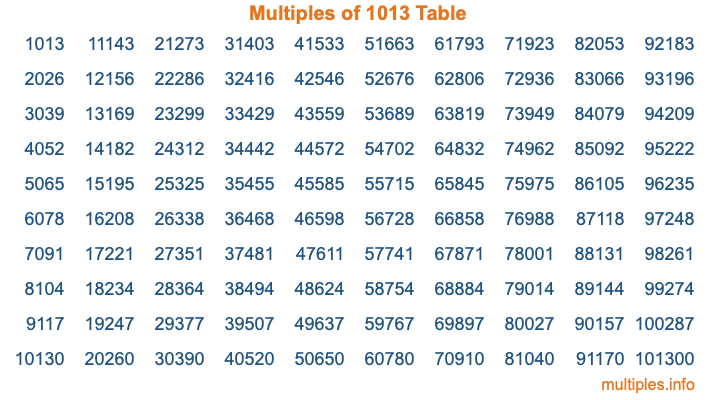Multiples of 1013Welcome to the Multiples of 1013 page. Here we will first teach you everything you will ever need to know about the multiples of 1013, and then give you a study guide summary of everything we taught you to make sure you remember it all. Use this page to look up facts and learn information about the multiples of 1013. This page will make you a multiples of one thousand thirteen expert!

Definition of Multiples of 1013
Multiples of 1013 are all the numbers that when divided by 1013 equal an integer. Each of the multiples of 1013 are called a multiple. A multiple of 1013 is created by multiplying 1013 by an integer.

Therefore, to create a list of multiples of 1013, you start with 1 multiplied by 1013, then 2 multiplied by 1013, then 3 multiplied by 1013, and so on for as long as you want. Thus, the list of the first five multiples of 1013 is 1013, 2026, 3039, 4052, and 5065. To see a larger list of multiples of 1013, see the printable image of Multiples of 1013 further down on this page. We also have a category where you can choose any nth multiple of 1013.

Multiples of 1013 Checker
The Multiples of 1013 Checker below checks to see if any number of your choice is a multiple of 1013. In other words, it checks to see if there is any number (integer) that when multiplied by 1013 will equal your number. To do that, we divide your number by 1013. If the the quotient is an integer, then your number is a multiple of 1013.

Is  a multiple of 1013?

Least Common Multiple of 1013 and ...
A Least Common Multiple (LCM) is the lowest multiple that two or more numbers have in common. This is also called the smallest common multiple or lowest common multiple and is useful to know when you are adding our subtracting fractions. Enter one or more numbers below (1013 is already entered) to find the LCM.

Check out our LCM Calculator if you need more details about the Least Common Multiple or if you need the LCM for different numbers for adding and subtraction fractions.

nth Multiple of 1013
As we stated above, 1013 is the first multiple of 1013, 2026 is the second multiple of 1013, 3039 is the third multiple of 1013, and so on. Enter a number below to find the nth multiple of 1013.

th multiple of 1013

Multiples of 1013 vs Factors of 1013
1013 is a multiple of 1013 and a factor of 1013, but that is where the similarities end. All postive multiples of 1013 are 1013 or greater than 1013. All positive factors of 1013 are 1013 or less than 1013.

Below is the beginning list of multiples of 1013 and the factors of 1013 so you can compare:

Multiples of 1013: 1013, 2026, 3039, 4052, 5065, etc.

Factors of 1013: 1, 1013

As you can see, the multiples of 1013 are all the numbers that you can divide by 1013 to get a whole number. The factors of 1013, on the other hand, are all the whole numbers that you can multiply by another whole number to get 1013.

It's also interesting to note that if a number (x) is a factor of 1013, then 1013 will also be a multiple of that number (x).

Multiples of 1013 vs Divisors of 1013
The divisors of 1013 are all the integers that 1013 can be divided by evenly. Below is a list of the divisors of 1013.

Divisors of 1013: 1, 1013

The interesting thing to note here is that if you take any multiple of 1013 and divide it by a divisor of 1013, you will see that the quotient is an integer.

Multiples of 1013 Table
Below is an image of the first 100 multiples of 1013 in a table. The table is in chronological order, column by column. The first column has the first ten multiples of 1013, the second column has the next ten multiples of 1013, and so on.The Multiples of 1013 Table is also referred to as the 1013 Times Table or Times Table of 1013. You are welcome to print out our table for your studies.

Negative Multiples of 1013
Although not often discussed or needed in math, it is worth mentioning that you can make a list of negative multiples of 1013 by multiplying 1013 by -1, then by -2, then by -3, and so on, to get the following list of negative multiples of 1013:

-1013, -2026, -3039, -4052, -5065, etc.

Multiples of 1013 Summary
Below is a summary of important Multiples of 1013 facts that we have discussed on this page. To retain the knowledge on this page, we recommend that you read through the summary and explain to yourself or a study partner why they hold true.

There are an infinite number of multiples of 1013.

A multiple of 1013 divided by 1013 will equal a whole number.

1013 divided by a factor of 1013 equals a divisor of 1013.

The nth multiple of 1013 is n times 1013.

The largest factor of 1013 is equal to the first positive multiple of 1013.

1013 is a multiple of every factor of 1013.

1013 is a multiple of 1013.

A multiple of 1013 divided by a divisor of 1013 equals an integer.

1013 divided by a divisor of 1013 equals a factor of 1013.

Any integer times 1013 will equal a multiple of 1013.

Multiples of a Number
Here you can get the multiples of another number, all with the same attention to detail as we did for multiples of 1013 on this page.

Multiples of
Multiples of 1014
Did you find our page about multiples of one thousand thirteen educational? Do you want more knowledge? Check out the multiples of the next number on our list!